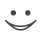# Get these flashcards, study & pass exams. For free! Even on iPhone/Android!

## Alli's Geometry Flashcards(: (25 Cards)

What Does The Segment Addition Postulate State?
If Three Points A, B, And C, Then AB + BC = AC.
Which Theorem States If The Two Angles Of One Triangle Are Congruent To Two Angles Of Another Triangle, Then The Third Angles Are Congruent?
Theorem 4-1.
What Is Postulate 4-3 (ASA)?
If Two Angles And The Included Side Of One Triangle Are Congruent To Two Angles And The Included Side Of Another Triangle, Then The Two Triangles Are Congruent.
Which Postulate States That If Three Sides Of One Triangle Are Congruent To The Three Sides Of Another Triangle, Then The Two Triangles Are Congruent?
Postulate 4-1 (SSS)
What Does The Theorem 4-2 State (SAS)?
If Two Sides And The Included Angle Of One Triangle Are Congruent To Two Sides And The Included Angle Of Another Triangle, Then The Two Triangles Are Congruent.
What Theorem States If A Transversal Intersects Two Parallel Lines Then Same-side Interior Angles Are Supplementary?
Same Side Interior Angles Theorem.
What Does Theorem 3-5 State?
If Two Lines Are Parallel To The Same Line, Then They Are Parallel To Each Other.
What Does The Triangle Angle-Sum Theorem State?
The Sum Of The Measures Of The Angles Of A Triangle Is 180.
What Theorem States That The Sum Of The Measures Of The Angles Of An n-gon Is (n-2)180?
Theorem 3-9: Polygon Angle-Sum Theorem.
What Is The Alternate Interior Angles Theorem?
If A Transversal Intersects Two Parallel Lines, Then The Alternate Interior Angles Are Congruent.
What Is The Triangle Midsegment Theorem?
If A Segment Joins The Midpoints Of Two Sides Of A Triangle, Then The Segment Is Parallel To The Third Side, And Is Half Its Length.
Which Theorem StatesThat The Lines That Contain The Altitudes Of A Triangle Are Concurrent?
Theorem 5-9.
What Theorem States If Two Angles Of A Triangle Are Not Congruent, Then The Longer Side Lies Opposite The Longer Side?
Theorem 5-11
What Does The Perpendicular Bisector Theorem State?
If A Point Is On The Perpendicular Bisector Of A Segment, Then It Is Equidistant From The Endpoints Of The Segment.
What Does Theorem 5-6 State?
The Perpendicular Bisectors Of The Sides Of A Triangle Are Concurrent At A Point Equidistant From The Vertices.
What Does Theorem 4-3 State?
If Two Sides Of A Triangle Are Congruent, Then The Angles Opposite Those Sides Are Congruent.
What Does Postulate 1-3 State?
If Two Planes Intersect, Then They Intersect In Exactly One Line.
What Is The Law Of Syllogism?
If p > q And q > r Are True Statements, Then p --> r Is A True Statement.
What Is Are The Properties Of Congruence?
Reflexive Property, Symmetric Property, And Transitive Property.
What Does Theorem 2-4 State?
All Right Angles Are Congruent.
What Is The Vertical Angles Theorem?
Vertical Angles Are Congruent.
What Is The Law Of Detatchment?
If A Conditional Is True And Its Hypothesis Occurs, Then Its Conclusion Is Guarenteed. In Symbolic Form: If p --> q Is A True Statement And p Is True, Then q Is True.
What Postulate States If Two Figures Are Congruent, Then Their Areas Are Equal?
Postulate 1-9.
Which Postulate States That The Area Of A Region Is The Sum Of The Areas Of Its Nonoverlapping Parts?
Postulate 1-10.
Which Postulate States Through Any Two Points There Is Exactly One Line?
Postulate 1-1.Flashcard set info:
Author: CoboCards-User
Main topic: Mathematics
Topic: Geometry
Published: 12.01.2011

Card tags:
All cards (25)
no tagsReport abuse